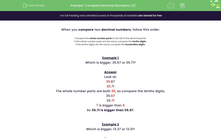# Compare Decimal Numbers (2)

In this worksheet, students must compare two decimal numbers up to two decimal places.Key stage:  KS 2

Curriculum topic:   Number: Fractions and Decimals

Curriculum subtopic:   Compare Decimals up to Two Places

Difficulty level:#### Worksheet Overview

When you compare two decimal numbers, follow this order:

1. Compare the whole number parts to the left of the decimal points.
2. If the whole number parts are the same, compare the tenths digits.
3. If the tenths digits are the same, compare the hundredths digits.

Example 1

Which is bigger, 35.67 or 35.71?

Look at:

35.67

35.71

The whole number parts are both 35, so compare the tenths digits.

35.67

35.71

7 is bigger than 6

So 35.71 is bigger than 35.67.

Example 2

Which is bigger, 13.37 or 13.31?

Look at:

13.37

13.31

The whole number parts are both 13, so compare the tenths digits.

13.37

13.31

The tenths digits are both 3, so compare the hundredths digits.

13.37

13.31

7 is bigger than 1

So 13.37 is bigger than 13.31.

Example 3

Which is bigger, 24.07 or 12.71?

Look at:

24.07

12.71

The whole number parts are 24 and 12.

24 is bigger than 12

So 24.07 is bigger than 12.71.

### What is EdPlace?

We're your National Curriculum aligned online education content provider helping each child succeed in English, maths and science from year 1 to GCSE. With an EdPlace account you’ll be able to track and measure progress, helping each child achieve their best. We build confidence and attainment by personalising each child’s learning at a level that suits them.

Get started